﻿ The Analyses of Large Displacement Pendulum Movement Using High-Speed Digital Image Correlation and Matlab/Simulink

### The Analyses of Large Displacement Pendulum Movement Using High-Speed Digital Image Correlation and ...

Martin Hagara, Róbert Huňady, František Trebuňa, Peter Pavelka

American Journal of Mechanical Engineering

## The Analyses of Large Displacement Pendulum Movement Using High-Speed Digital Image Correlation and Matlab/Simulink

Martin Hagara1,, Róbert Huňady1, František Trebuňa1, Peter Pavelka1

1Department of Applied Mechanics and Mechanical Engineering, Technical University of Košice, Faculty of Mechanical Engineering, Košice, Slovakia

### Abstract

This contribution deals with the possibility of using high-speed digital image correlation system in motion analysis. In the first part the principle and methodology of high-speed measurements is briefly introduced. The paper also describes the analysis by which the minimal sampling frequency of cameras necessary for registration of increasing velocity of the investigated object was determined. In the second part of the contribution the motion of a simple pendulum is described analytically. In the third part the experimental motion analysis of a simple pendulum was performed using high-speed digital image correlation system Q-450 Dantec Dynamics, by which its behavior was investigated and finally, the obtained results were verified using numerical simulation in Matlab/Simulink.

• Martin Hagara, Róbert Huňady, František Trebuňa, Peter Pavelka. The Analyses of Large Displacement Pendulum Movement Using High-Speed Digital Image Correlation and Matlab/Simulink. American Journal of Mechanical Engineering. Vol. 4, No. 7, 2016, pp 406-412. http://pubs.sciepub.com/ajme/4/7/32
• Hagara, Martin, et al. "The Analyses of Large Displacement Pendulum Movement Using High-Speed Digital Image Correlation and Matlab/Simulink." American Journal of Mechanical Engineering 4.7 (2016): 406-412.
• Hagara, M. , Huňady, R. , Trebuňa, F. , & Pavelka, P. (2016). The Analyses of Large Displacement Pendulum Movement Using High-Speed Digital Image Correlation and Matlab/Simulink. American Journal of Mechanical Engineering, 4(7), 406-412.
• Hagara, Martin, Róbert Huňady, František Trebuňa, and Peter Pavelka. "The Analyses of Large Displacement Pendulum Movement Using High-Speed Digital Image Correlation and Matlab/Simulink." American Journal of Mechanical Engineering 4, no. 7 (2016): 406-412.

 Import into BibTeX Import into EndNote Import into RefMan Import into RefWorks

### 1. Introduction

A system is said to be linear or nonlinear depending on the force response characteristic of the system. Let us consider a simple mechanical system of undamped, unforced pendulum, which scheme is depicted in Figure 1.Download asVeiw figureFigures index
Figure 1. Scheme of simple pendulum

The motion of such pendulum can be expressed by the differential equation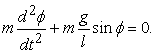(1)

If the pendulum were damped, equation (1) changes to the following form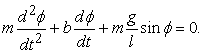(2)

where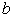is a damping constant. Eq. (2) says, that simple pendulum has an approximately linear behavior for very small angles of displacement, however, on the other hand it indicates inherently nonlinear behavior in case, if the initial angular position becomes larger.

Find a solution of such nonlinear equation using analytical approach is quite a difficult task. Therefore, a process, well-known as linearization (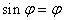), is used.

While in linear oscillations all the periods between course maxima are constant, for the nonlinear one it is characteristic, that the amount of period is changing during motion [1, 2].

Our task was to investigate the behavior of simple pendulum experimentally using a noncontact full-field method of high-speed digital image correlation and the obtained results compare to the results acquired numerically.

### 2. High-speed Digital Image Correlation

High-speed digital image correlation is a modern experimental technique serving primarily for the investigation of displacement fields, vibration analysis, fatigue tests or motion analysis [3, 4, 5, 6]. For the measurement it utilizes stereoscopically arranged CCD cameras, by which an investigated object is captured during its motion (Figure 2).Download asVeiw figureFigures index
Figure 2. Stereoscopically arranged CCD cameras for 3D measurement

By the evaluation, which is called correlation, the captured images are compared (correlated) among each other. For a reason that the images are correlated along small picture elements called facets, there is a necessity to create a random black and white pattern on the investigated object surface (Figure 3). Created pattern copies the object contour and moves with it together. The results of the analysis are then in a form of 3D contour as well as displacement fields.Download asVeiw figureFigures index
Figure 3. Random black and white pattern

For a reason that CCD cameras have a limited internal memory, it is necessary to know the relation between sampling frequency of the cameras and the highest object velocity, when the random pattern remained still sharp enough to be correlated. The result of the experiment is a dependence depicted in Figure 4.Download asVeiw figureFigures index
Figure 4. Dependence between minimal sampling frequency of the cameras and maximal velocity of a point, which can be recorded at given frequency

### 3. Experimental Motion Analysis of a Simple Pendulum Using High-Speed Digital Image Correlation

The motion analysis was performed on a simple pendulum which consists of elements depicted in Figure 5. The length from the rotation axis to the bob center of mass was 125 mm. The weight of the pendulum was 0.13 kg.Download asVeiw figureFigures index
Figure 5. Scheme of investigated pendulum with its dimensions

In Figure 6 a reference image, captured by one of the cameras, can be seen. It can be clearly seen that two random pattern were created on the investigated object. The first one was created in the stationary middle part of the bearings for the definition of coordinate system. The second one was made for investigation of chosen bob point motion. Both of them were printed on a vinyl foil and attached to corresponding parts of pendulum.Download asVeiw figureFigures index
Figure 6. Reference image captured by one of the correlation system cameras

The measurement was performed when pendulum was deflected from its equilibrium position about 60° and freely dropped to move. Realization of the measurement was done using cameras Phantom Speed Sense 9070 with spatial resolution of 1 MPx and internal memory of 16 GB. For the relatively high speed of the pendulum points the sampling frequency of the cameras was set to Fs = 2500 fps, i.e. between each step exists a time interval of Δt = 1/Fs = 0.0004 s. The mentioned cameras allowed capture maximally 16205 images, what means that the acquisition time was equal to 6.482 s.

In Figure 7 the contour of investigated pendulum parts evaluated by the system Q-450 can be seen. In these parts the rectangular right-handed Cartesian coordinate system xy and an investigated point were defined. The location of the investigated point corresponds to the bob center of mass.Download asVeiw figureFigures index
Figure 7. Evaluated pendulum parts with defined coordinate system and investigated point

The time dependences of investigated point displacements in x and y direction were imported into program Matlab, in which they were numerically processed. For the computation of velocity and acceleration of the investigated point it is possible to use kinematical principles valid for the motion of a point in Cartesian coordinate system. If the obtained displacements were continuous functions of time the following relations for the point velocity computation could be use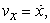(3)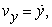(4)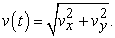(5)

For the computation of point acceleration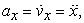(6)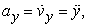(7)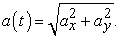(8)

The angular velocity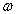and angular acceleration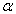of the pendulum bob can be found by sequential differentiation of angular position φ by time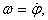(9)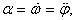(10)

where angular position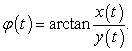(11)

Taking into account that the obtained displacements are in a form of discrete values, for the computation of mentioned quantities a method of numerical differentiation has to be used . Using this method, the differentiation of each function f(t) by time can be expressed as follows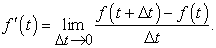(12)

If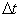is considered as very small amount, than relation (12) can be replaced by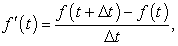(13)

The obtained courses of investigated point quantities can be found in Figure 8 – Figure 16.Download asVeiw figureFigures index
Figure 8. Time course of displacements in x direction obtained by DICDownload asVeiw figureFigures index
Figure 9. Time course of displacements in y direction obtained by DICDownload asVeiw figureFigures index
Figure 10. Trajectory of the point during pendulum motion obtained by DICDownload asVeiw figureFigures index
Figure 11. Time course of the point velocity obtained by DICDownload asVeiw figureFigures index
Figure 12. Time course of the point acceleration obtained by DICDownload asVeiw figureFigures index
Figure 13. Time course of the point angular position obtained by DICDownload asVeiw figureFigures index
Figure 14. Time course of the point angular velocity obtained by DICDownload asVeiw figureFigures index
Figure 15. Phase diagram of the point motion obtained by DICDownload asVeiw figureFigures index
Figure 16. Time course of the point angular acceleration obtained by DIC

The motion of the pendulum with same parameters was modeled in Matlab/Simulink. The missing parameter of damping constant b was calculated from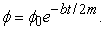(14)

For the solution of the differential equation (2) it was necessary to create in Matlab/Simulink a numerical model, which scheme is depicted in Figure 17. The damping constant was set to b = 0.014 kg.s-1. In Gain1 from the Simulink scheme the ratio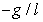was set, then in Gain the ratio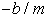was set and finally in Integrator1 the initial conditions were set to angular position equal to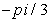.Download asVeiw figureFigures index
Figure 17. Scheme for the creation of numerical model for computation of differential equation of the pendulum motion used in Matlab/Simulink

The comparison of obtained time courses of investigated point angular position can be seen in Figure 18. As can be seen, the shown plots confirm a good correspondence between the results obtained experimentally by DIC and numerically by Matlab/Simulink.Download asVeiw figureFigures index
Figure 18. Temporal plots of investigated point angular position obtained by DIC and Matlab/Simulink

### 4. Summary

Digital image correlation is a modern technique, which has a wide range of applications in experimental mechanics. Its high-speed configuration can be used in the motion analysis. For this application it is necessary to know the minimal sampling frequency needed for acquiring images with sufficient quality and sharpness. For the accomplishment of this condition it was assessed that the minimal sampling frequency should be set approximately 130-135 times higher than maximal velocity of an investigated object point.

The contribution describes the methodology for the investigation of kinematic quantities of simple pendulum point, when the initial angular position of the pendulum is large and thus its motion has a nonlinear behavior. The universality of the described noncontact full-field method makes this technique very effective in experimental mechanics’ applications. Its main advantages are material and geometrical independence, flexible measurement area, spatial visualization of the results, possibilities of measurements in arbitrary location of observed area and simple processing of obtained data.

The results obtained experimentally via high-speed digital image correlation system correspond well to the results acquired numerically using Matlab/Simulink.

### Acknowledgements

The paper is the result of the projects implementation VEGA 1/0393/14 and VEGA 1/0731/16.

### References

  Soukup, J. and Svoboda, M., Vertical Oscillation of a Simplified Model a Mechanical System, Journal of Applied Nonlinear Dynamics, 3(4), 347-358, December 2014.In article View Article  Svoboda, M., and Soukup, J., The Influence of Geometry, Manufacturing Asymmetry and Asymmetric Excitation on Vertical Vibration of a Mechanical System, Applied Mechanics and Materials, 302, 429-434, 2013.In article View Article  Trebuňa, F., and Hagara, M., Experimental modal analysis performed by high-speed digital image correlation system, Measurement: Journal of the International Measurement Confederation, 50(4), 78-85, 2014.In article  Wang, Z., Nguyen, H. and Quisberth, J., Audio extraction from silent high-speed video using an optical technique, Optical Engineering, 53(11), 1-3, 2014.In article View Article  Huňady, R., Hagara, M. and Schrotter, M., Using high-speed digital image correlation to determine the damping ratio, Procedia Engineering, 48, 242-249, 2012.In article View Article  Baqersad, J., Poozesh, P., Niezrecki, Ch. and Avitabile, P., Photogrammetry and optical methods in structural dynamics – A review, Mechanical Systems and Signal Processing, (In Press), 2016.In article  B. Rizwan, Introduction to Numerical Analysis using Matlab, Infinity Science Press, 2008.In article